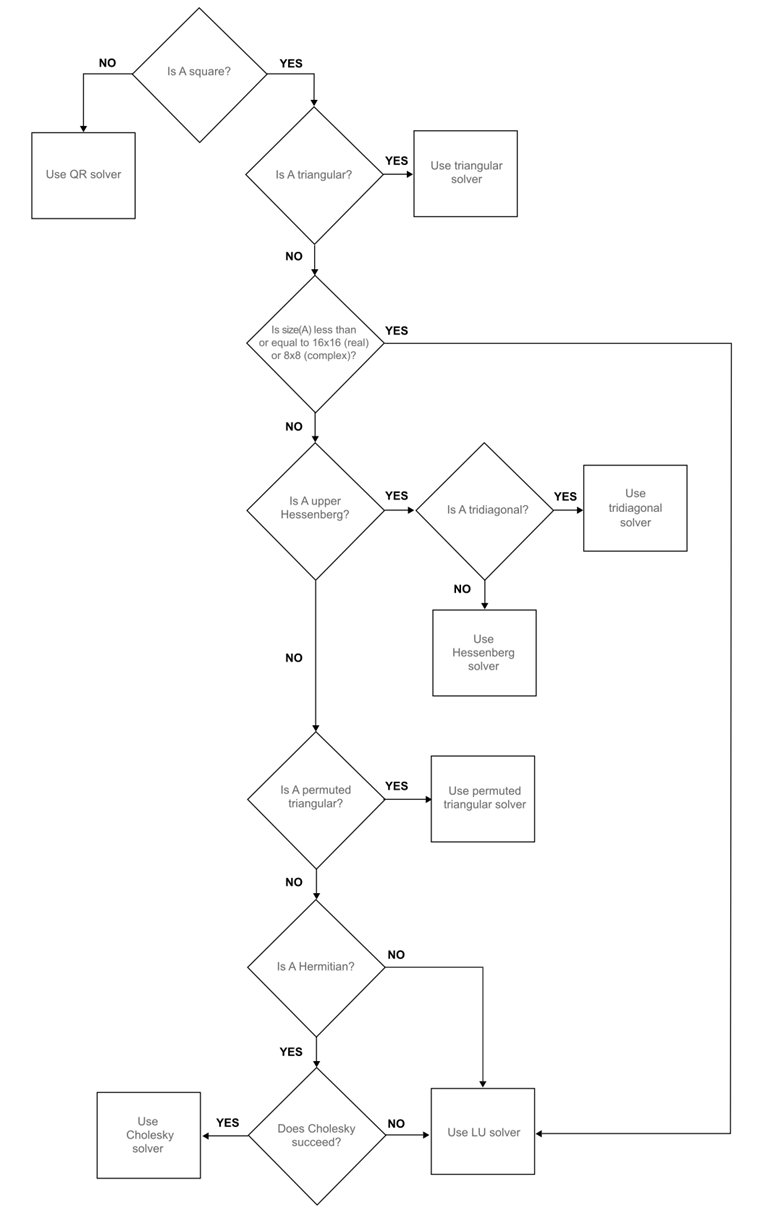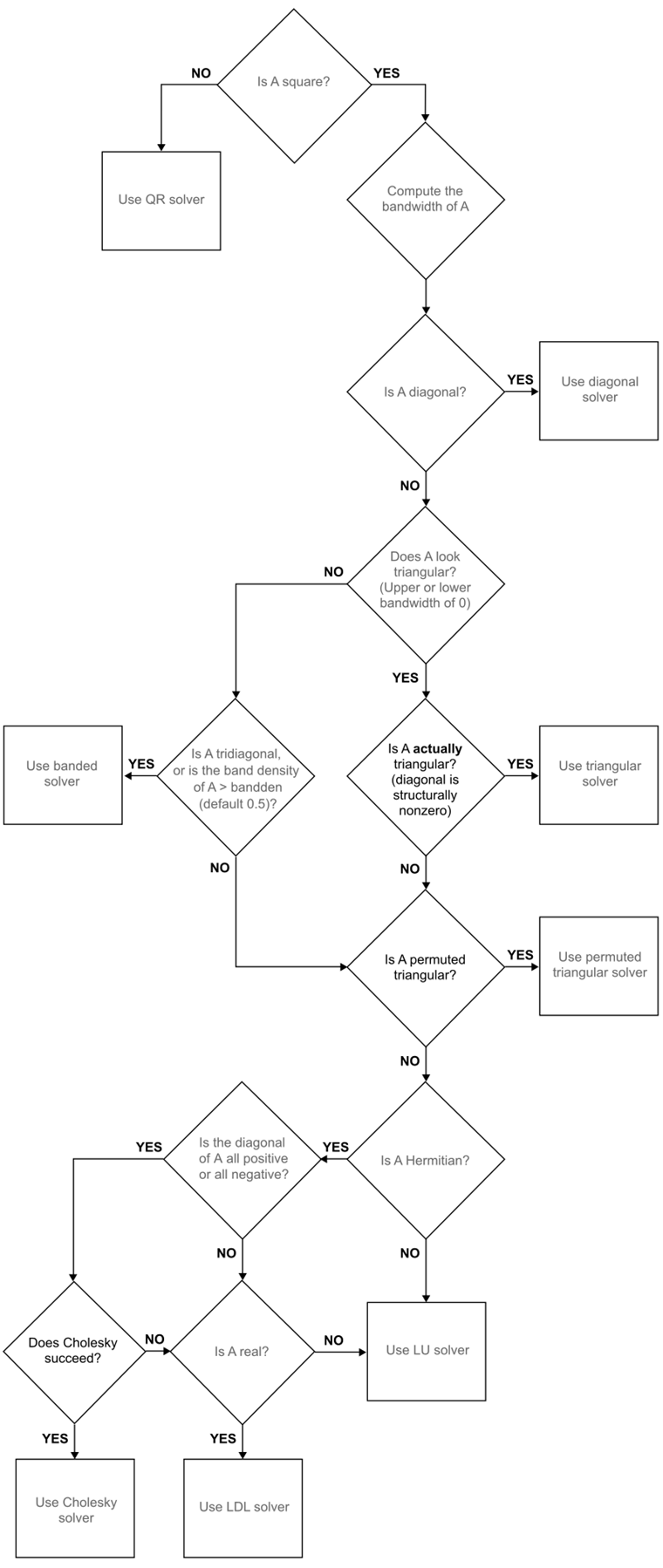# mldivide, \

Solve systems of linear equations Ax = B for x

## Syntax

``x = A\B``
``x = mldivide(A,B)``

## Description

example

````x = A\B` solves the system of linear equations ```A*x = B```. The matrices `A` and `B` must have the same number of rows. MATLAB® displays a warning message if `A` is badly scaled or nearly singular, but performs the calculation regardless.If `A` is a scalar, then `A\B` is equivalent to `A.\B`.If `A` is a square `n`-by-`n` matrix and `B` is a matrix with `n` rows, then ```x = A\B``` is a solution to the equation `A*x = B`, if it exists.If `A` is a rectangular `m`-by-`n` matrix with `m ~= n`, and `B` is a matrix with `m` rows, then `A`\`B` returns a least-squares solution to the system of equations `A*x= B`.```
``` `x = mldivide(A,B)` is an alternative way to execute `x` `=` `A`\`B`, but is rarely used. It enables operator overloading for classes.```

## Examples

collapse all

Solve a simple system of linear equations, `A*x = B`.

```A = magic(3); B = [15; 15; 15]; x = A\B```
```x = 3×1 1.0000 1.0000 1.0000 ```

Solve a linear system of equations `A*x = b` involving a singular matrix, `A`.

```A = magic(4); b = [34; 34; 34; 34]; x = A\b```
```Warning: Matrix is close to singular or badly scaled. Results may be inaccurate. RCOND = 1.306145e-17. ```
```x = 4×1 1.5000 2.5000 -0.5000 0.5000 ```

When `rcond` is between `0` and `eps`, MATLAB® issues a nearly singular warning, but proceeds with the calculation. When working with ill-conditioned matrices, an unreliable solution can result even though the residual `(b-A*x)` is relatively small. In this particular example, the norm of the residual is zero, and an exact solution is obtained, although `rcond` is small.

When `rcond` is equal to `0`, the singular warning appears.

```A = [1 0; 0 0]; b = [1; 1]; x = A\b```
```Warning: Matrix is singular to working precision. ```
```x = 2×1 1 Inf ```

In this case, division by zero leads to computations with `Inf` and/or `NaN`, making the computed result unreliable.

Solve a system of linear equations, `A*x = b`.

```A = [1 2 0; 0 4 3]; b = [8; 18]; x = A\b```
```x = 3×1 0 4.0000 0.6667 ```

Solve a simple system of linear equations using sparse matrices.

Consider the matrix equation `A*x = B`.

```A = sparse([0 2 0 1 0; 4 -1 -1 0 0; 0 0 0 3 -6; -2 0 0 0 2; 0 0 4 2 0]); B = sparse([8; -1; -18; 8; 20]); x = A\B```
```x = (1,1) 1.0000 (2,1) 2.0000 (3,1) 3.0000 (4,1) 4.0000 (5,1) 5.0000 ```

## Input Arguments

collapse all

Operands, specified as vectors, full matrices, or sparse matrices. `A` and `B` must have the same number of rows.

• If `A` or `B` has an integer data type, the other input must be scalar. Operands with an integer data type cannot be complex.

Data Types: `single` | `double` | `int8` | `int16` | `int32` | `int64` | `uint8` | `uint16` | `uint32` | `uint64` | `logical` | `char`
Complex Number Support: Yes

## Output Arguments

collapse all

Solution, returned as a vector, full matrix, or sparse matrix. If `A` is an `m`-by-`n` matrix and `B` is an `m`-by-`p` matrix, then `x` is an `n`-by-`p` matrix, including the case when `p==1`.

If `A` has full storage, `x` is also full. If `A` is sparse, then `x` has the same storage as `B`.

## Tips

• The operators `/` and `\` are related to each other by the equation ```B/A = (A'\B')'```.

• If `A` is a square matrix, then `A\B` is roughly equal to `inv(A)*B`, but MATLAB processes `A\B` differently and more robustly.

• If the rank of `A` is less than the number of columns in `A`, then `x = A\B` is not necessarily the minimum norm solution. You can compute the minimum norm least-squares solution using `x = lsqminnorm(A,B)` or `x = pinv(A)*B`.

• Use `decomposition` objects to efficiently solve a linear system multiple times with different right-hand sides. `decomposition` objects are well-suited to solving problems that require repeated solutions, since the decomposition of the coefficient matrix does not need to be performed multiple times.

## Algorithms

collapse all

The versatility of `mldivide` in solving linear systems stems from its ability to take advantage of symmetries in the problem by dispatching to an appropriate solver. This approach aims to minimize computation time. The first distinction the function makes is between full (also called “dense”) and sparse input arrays.

### Algorithm for Full Inputs

The flow chart below shows the algorithm path when inputs `A` and `B` are full.### Algorithm for Sparse Inputs

If `A` is full and `B` is sparse then `mldivide` converts `B` to a full matrix and uses the full algorithm path (above) to compute a solution with full storage. If `A` is sparse, the storage of the solution `x` is the same as that of `B` and `mldivide` follows the algorithm path for sparse inputs, shown below.Gilbert, John R., and Tim Peierls. “Sparse Partial Pivoting in Time Proportional to Arithmetic Operations.” SIAM Journal on Scientific and Statistical Computing 9, no. 5 (September 1988): 862–874. https://doi.org/10.1137/0909058.

 Anderson, E., ed. LAPACK Users’ Guide. 3rd ed. Software, Environments, Tools. Philadelphia: Society for Industrial and Applied Mathematics, 1999. https://doi.org/10.1137/1.9780898719604.

 Davis, Timothy A. "Algorithm 832: UMFPACK V4.3 – an unsymmetric-pattern multifrontal method." ACM Transactions on Mathematical Software 30, no. 2 (June 2004): 196–199. https://doi.org/10.1145/992200.992206.

 Duff, Iain S. “MA57---a Code for the Solution of Sparse Symmetric Definite and Indefinite Systems.” ACM Transactions on Mathematical Software 30, no. 2 (June 2004): 118–144. https://doi.org/10.1145/992200.992202.

 Davis, Timothy A., John R. Gilbert, Stefan I. Larimore, and Esmond G. Ng. “Algorithm 836: COLAMD, a Column Approximate Minimum Degree Ordering Algorithm.” ACM Transactions on Mathematical Software 30, no. 3 (September 2004): 377–380. https://doi.org/10.1145/1024074.1024080.

 Amestoy, Patrick R., Timothy A. Davis, and Iain S. Duff. “Algorithm 837: AMD, an Approximate Minimum Degree Ordering Algorithm.” ACM Transactions on Mathematical Software 30, no. 3 (September 2004): 381–388. https://doi.org/10.1145/1024074.1024081.

 Chen, Yanqing, Timothy A. Davis, William W. Hager, and Sivasankaran Rajamanickam. “Algorithm 887: CHOLMOD, Supernodal Sparse Cholesky Factorization and Update/Downdate.” ACM Transactions on Mathematical Software 35, no. 3 (October 2008): 1–14. https://doi.org/10.1145/1391989.1391995.

 Davis, Timothy A. “Algorithm 915, SuiteSparseQR: Multifrontal Multithreaded Rank-Revealing Sparse QR Factorization.” ACM Transactions on Mathematical Software 38, no. 1 (November 2011): 1–22. https://doi.org/10.1145/2049662.2049670.